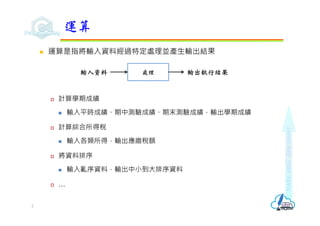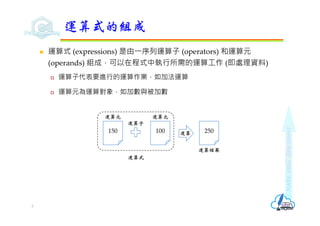Successfully reported this slideshow.×

# C語言運算式和運算子

### C語言運算式和運算子

1. 1. 運算式和運算子  運算作業  指定運算  算術運算  遞增遞減運算  比較運算  邏輯運算子  if敘述  位元運算  資料型別轉換  運算子的優先序 與結合性 Revised on July 25, 2021
2. 2. Make each day count  運算是指將輸入資料經過特定處理並產生輸出結果  計算學期成績  輸入平時成績、期中測驗成績、期末測驗成績，輸出學期成績  計算綜合所得稅  輸入各類所得，輸出應繳稅額  將資料排序  輸入亂序資料，輸出中小到大排序資料  … 運算 2 處理 輸入資料 輸出執行結果
3. 3. Make each day count  運算式 (expressions) 是由⼀序列運算子 (operators) 和運算元 (operands) 組成，可以在程式中執行所需的運算工作 (即處理資料)  運算子代表要進行的運算作業，如加法運算  運算元為運算對象，如加數與被加數 運算式的組成 3 150 運算元 100 運算元 250 運算結果 運算子 運算 運算式
4. 4. Make each day count  C 語言的運算子依運算元個數可以分成三種  單元運算子 (unary operator)：只需⼀個運算元，例如：負號、遞增、遞增  -15  10++  二元運算子 (binary operator)：需要有位在左右的兩個運算元，C 語言的 運算子大部分都是二元運算子  5 + 10  10 * 2  三元運算子 (ternary operator)：需要3個運算元，C 語言只有⼀種三元運算 子「? :」用來建立條件運算式，詳見單元5說明  max = (a > b)? a : b; C語言的運算子 4
5. 5. Make each day count  指定運算式 (assignment expressions) 就是指定敘述，將右邊運算元 或運算式運算結果，存入位在左邊的變數 int x = 10, y = 20, temp; printf("x=%d, y=%dn", x, y); temp = x; x = y; y = temp; printf("x=%d, y=%dn", x, y); 指定運算子 5
6. 6. Make each day count  算術運算子 (arithmetic operators) 可以建立數學的算術運算式 (arithmetic expressions) 算術運算子 1/2 6 運算子 說明 運算式範例 - 負號 -7 + 正號 +7 * 乘法 7*3 / 除法 7/3 % 餘數 7%3 + 加法 7+3 - 減法 7-3
7. 7. Make each day count int num1 = 7, num2 = 3; printf("%d+%d=%dn", num1, num2, num1 + num2); printf("%d-%d=%dn", num1, num2, num1 - num2); printf("%d*%d=%dn", num1, num2, num1 * num2); printf("%d/%d=%dn", num1, num2, num1 / num2); printf("%d%%%d=%dn", num1, num2, num1 % num2); 算術運算子 2/2 7
8. 8. Make each day count  圓球體表面積公式為 4𝜋𝑟 ，圓球體體積公式為 𝜋𝑟 ，圓周率𝜋以 3.14159計算，設計程式完成以下功能  輸入圓球半徑 (浮點數)，計算並顯示圓球體表面積及體積 (顯示到小數點 第2位) Lab1 計算球體表面積及體積 1/2 8
9. 9. Make each day count #include <stdio.h> void homework1(){ const float PI = 3.14159; float radius, surface, volume; printf("輸入半徑值(公分)："); scanf("%f", &radius); surface = 4 * PI * radius * radius; volume = 4 / 3.0 * PI * radius * radius * radius; // 注意：不能使用4/3 printf("圓球表面積為%0.2f平方公分n", surface); printf("圓球體積為%0.2f立方公分n", volume); } Lab1 計算球體表面積及體積 2/2 9
10. 10. Make each day count  計算體脂肪 BMI 的公式是「體重÷ (身高×身高)」，身高單位公尺， 體重單位公斤，設計程式完成以下功能  輸入身高 (公分) 及體重 (公斤)，計算並顯示 BMI 值 (顯示到小數點第1位) Lab2 BMI計算程式 1/2 10
11. 11. Make each day count #include <stdio.h> void bmi(){ float weight, height; float bmi; printf("輸入身高(公分)："); scanf("%f", &height); printf("輸入體重(公斤)："); scanf("%f", &weight); height = height / 100.0; bmi = weight / (height * height); printf("體脂肪BMI值為%0.1fn", bmi); } Lab2 BMI計算程式 2/2 11
12. 12. Make each day count  遞增運算子 (++) 和遞減運算子 (--) 是位在變數運算元之前 (稱為前置運 算)或之後 (稱為後置運算) 來建立運算式，可以執行變數值加1或減1的 運算 遞增和遞減運算子 1/2 12 運算子 說明 運算式範例 說明 ++ 遞增運算 x++; 後置 (postfix) 遞增運算 ++x; 前置 (prefix) 遞增運算 -- 遞減運算 x--; 後置遞減運算 --x; 前置遞減運算
13. 13. Make each day count  對變數自身而言，前置遞增或後置遞增結果相同，前置遞減或後置遞 減結果也相同，但若混合其他運算子時，就要注意變數取值順序 int x = 10; int y; y = x++; //先將x值傳給y後，x值再遞增。y值為10，x值為11 y = ++x; //x先遞增後，再將x值傳給y。y值為12，x值為12 y = x--; //先將x值傳給y後，x值再遞減。y值為12，x值為11 y = --x; //x先遞減後，再將x值傳給y。y值為10，x值為10 遞增和遞減運算子 2/2 13
14. 14. Make each day count  比較運算子 (Comparison Operators) 用來判斷二運算元之大小關係， 當回傳值為1，表示比較結果成立 (true)；回傳值為0時，表示比較結 果不成立 (false)。通常搭配條件敘述使用 比較運算子 1/2 14 運算子 說明 運算式範例 != 不等於 x != 10 < 小於 x < 10 <= 小於或等於 x <= 10 == 等於 x == 10 > 大於 x > 10 >= 大於或等於 x >= 10
15. 15. Make each day count int num1 = 7, num2 = 9; printf("%d != %d比較運算之結果為%dn", num1, num2, num1 != num2); printf("%d < %d比較運算之結果為%dn", num1, num2, num1 < num2); printf("%d <= %d比較運算之結果為%dn", num1, num2, num1 <= num2); printf("%d == %d比較運算之結果為%dn", num1, num2, num1 == num2); printf("%d > %d比較運算之結果為%dn", num1, num2, num1 > num2); printf("%d >= %d比較運算之結果為%dn", num1, num2, num1 >= num2); 比較運算子 2/2 15
16. 16. Make each day count  邏輯運算子用來將個別的條件運算式組合成複雜的條件運算式。通常 搭配條件敘述使用 邏輯運算子 16 運算子 說明 運算式範例 ! 反條件 !(a > 60) && 左右條件要同時成立 a >= 70 && a < 80 || 左右條件任⼀個成立 a < 70 || a >= 80
17. 17. Make each day count  if 敘述會依據條件運算式的結果，決定是否執行程式敘述，語法如下： if (條件運算式){ 程式區塊; }  如果條件運算式的結果為 true，就執行程式敘述；否則就跳過程式敘述  如果程式區塊只有單行敘述，則可以省略大括號 if敘述 1/2 17 條件運算式 程式區塊 true false
18. 18. Make each day count int score; printf("輸入成績："); scanf("%d", &score); if (score >= 60) printf("成績及格n"); if敘述 2/2 18
19. 19. Make each day count  ⽇常生活的二選⼀條件敘述是⼀種二分法，可以將⼀個集合分成二種 互斥的群組  超過60分屬於成績及格群組；反之為不及格群組  身高超過120公分是購買全票的群組；反之是購買半票的群組 if/else二選一條件敘述 1/3 19
20. 20. Make each day count  if條件敘述可以加上else敘述，變成二選⼀條件敘述，語法如下： if (條件運算式){ 程式區塊1; //條件成立執行的程式碼 } else { 程式區塊2; //條件不成立執行的程式碼 }  如果條件運算式成立true，就執行程式敘述1；不成立false，就執行程式敘 述2  如果程式區塊只有單行敘述，則可以省略大括號 if/else二選一條件敘述 2/3 20 條件運算式 程式區塊2 程式區塊1 true false
21. 21. Make each day count int score; printf("輸入成績："); scanf("%d", &score); if (score >= 60) printf("成績及格n"); else printf("成績不及格n"); if/else二選一條件敘述 3/3 21
22. 22. Make each day count  if/else敘述可繼續串加if/else敘述，變成多選⼀條件敘述，語法如下： if (條件1){ 程式區塊1; //條件1成立時，執行的程式碼 } else if (條件2){ 程式區塊2; //條件1不成立且條件2成立時，執行的程式碼 } else { 程式區塊3; //條件1與條件2皆不成立時，執行的程式碼 } if/else if多選一條件敘述 1/2 22 條件1 程式區塊3 程式區塊1 true false 條件1 true 程式區塊2 false
23. 23. Make each day count void test_elseif(){ int score; char grade; printf("輸入成績："); scanf("%d", &score); if(score >= 90) grade = 'A'; else if(score >= 80) grade = 'B'; else if(score >= 70) grade = 'C'; else if(score >= 60) grade = 'D'; else grade = 'F'; printf("成績等第%cn", grade); grade = (score >= 90)? 'A' : (score >= 80? 'B' : (score >= 70? 'C' : (score >= 60? 'D' : 'F'))); printf("成績等第%cn", grade); } if/else if多選一條件敘述 2/2 23
24. 24. Make each day count  設計程式完成以下功能： 輸入性別、身高 (cm) 及體重 (kg)，F 表女性 (female)，M 代表男性 (male)， 自動計算標準體重，並判斷體重狀況  標準體重計算方式  男性：(身高cm - 80)*70%  女性： (身高cm - 70)*60%  體重判定條件  在標準體重正負 10% 之間為正常體重  在標準體重正 10%~20% 之間為體重過重  在標準體重負 10%~20% 之間為體重過輕  超過標準體重正 20% 以上為肥胖  低於標準體重負 20% 以上為體重不足 Lab3 體重評估 1/4 24
25. 25. Make each day count Lab3 體重評估 2/4 25
26. 26. Make each day count void weight(){ float height, weight; float std_weight; char gender; printf("輸入性別(F代表女性，M代表男性)："); scanf("%c", &gender); printf("輸入身高(cm)："); scanf("%f", &height); printf("輸入體重(kg)："); scanf("%f", &weight); if (gender == 'F') std_weight = (height - 70) * 0.6; else std_weight = (height - 80) * 0.7; Lab3 體重評估 3/4 26
27. 27. Make each day count printf("標準體重是%0.1f公斤，你的體重是%0.1f公斤，為", std_weight, weight); if (weight >= std_weight * 0.9 && weight <= std_weight * 1.1) printf("正常體重n"); if (weight > std_weight * 1.1 && weight <= std_weight * 1.2) printf("體重過重n"); if (weight < std_weight * 0.9 && weight >= std_weight * 0.8) printf("體重過輕n"); if (weight > std_weight * 1.2) printf("肥胖n"); if (weight < std_weight * 0.8) printf("體重不足n"); } Lab3 體重評估 4/4 27
28. 28. Make each day count  位元運算子 (bitwise operators) 是對資料進行逐位元 (bitwise) 運算  位元左移運算，最高有效位元（most significant bit，MSB) 被移出(消失)， 最低有效位元（least significant bit，LSB) 補 0  位元右移運算，最高有效位補符號位元，最低有效位元被移出(消失) 位元運算子 1/2 28 運算子 說明 運算式範例 & 位元AND X & 10 << 位元左移 x << 1 >> 位元右移 x >> 1 ^ 位元XOR x ^ 0xF | 位元OR x | 0xF ~ 位元NOT ~x
29. 29. Make each day count short num = 0b01110101; printf("%#x & 0xff = %#xn", num, num & 255); printf("%#x << 1 = %#xn", num, num << 1); printf("%#x >> 1 = %#xn", num, num >> 1); printf("%#x ^ 0xff = %#xn", num, num ^ 255); printf("%#x | 0xff = %#xn", num, num | 255); printf("~%#x = %#xn", num, ~num); 位元運算子 2/2 29
30. 30. Make each day count  複合運算子 (Compound Operators) 同時執行『算術運算子或位元運 算子』及『指定運算子』兩件工作 複合指定運算子 30 運算子 範例 對等運算式 說明 += x += y; x = x + y; 加法 -= x -= y; x = x - y; 減法 *= x *= y; x = x * y; 乘法 /= x /= y; x = x / y; 除法 %= x %= y; x = x % y; 餘數 &= x &= y; x = x & y; 位元AND |= x |= y; x = x | y; 位元OR ^= x ^= y; x = x ^ y; 位元XOR >>= x >>= y; x = x >> y; 右移 <<= x <<= y; x = x << y; 左移
31. 31. Make each day count  sizeof是⼀種單元運算子，可以取得指定變數或資料型別所佔用記憶體 空間的位元組數  整數常數值 = sizeof(資料型別名稱);  整數常數值 = sizeof 變數名稱; sizeof運算子 1/2 31
32. 32. Make each day count int nums; printf("sizeof char type = %dn", sizeof(char)); printf("sizeof short int type = %dn", sizeof(short int)); printf("sizeof int type = %dn", sizeof(int)); printf("sizeof float type = %dn", sizeof(float)); printf("sizeof double type = %dn", sizeof(double)); printf("sizeof long double type = %dn", sizeof(long double)); printf("sizeof integer array variable nums = %dn", sizeof nums); sizeof運算子 2/2 32
33. 33. Make each day count  逗號運算子，是用來分隔敘述的，其運算優先權最低 int i = 1, j = 2; ++i, ++j; 逗號運算子 33
34. 34. Make each day count  程式設計時，常會有不同型別的資料進行運算，如果不注意型別轉換 (type cast)，很容易造成程式的錯誤  不同型別的變數進行指定運算時，編譯器會自動進行型別轉換  提升 (少指定給多) 將較少位元的型別指定給較多位元的型別時，會將較少位元的資料提升 為較多位元的型別 int n = 2; double m; m = n; //m=2.0 資料型別的轉換 1/3 34
35. 35. Make each day count  截斷 (多指定給少) 將較多位元的型別資料指定給較少位元的型別變數，會捨棄較多位元的部 份內容 int sum; int x = 2; float y = 0.6; sum = x + y; //無條件捨棄，sum=2 sum = x + y + 0.5; //四捨五入，sum=3  不同型別的變數進行指定以外的運算時，結果的型別會以較多位元者 為主 1/2結果為0 int/int → int 1/2.0結果為0.5 int/float → float 1.0/2結果為0.5 float/int → float 1.0/2.0結果為0.5 float/float → float 資料型別的轉換 2/3 35
36. 36. Make each day count  當需要不同型別的資料進行運算時，最好使用「型別轉換運算子」 (cast operator)，在運算式強迫轉換資料型別  (型別名稱) 運算式或變數 printf("3 / 2 = %dn", 3 / 2); printf("3.0 / 2 = %0.2fn", 3.0 / 2); printf("(float)3 / 2 = %0.2fn", (float)3 / 2); printf("(float)(3 / 2) = %0.2fn", (float)(3 / 2)); 資料型別的轉換 3/3 36
37. 37. Make each day count  當運算式使用多個運算子時，會依照運算子預設的優先順序 (precedence) 進行運算 y = b * 2 + 3;  「*」乘法運算子優先序大於「+」加法運算子及「=」指定運算子，所以會 先計算 b * 2 的值  「+」加法運算子優先序大於「=」指定運算子，所以計算 b * 2 + 3 之後的 值才會指定給 y 優先序和結合性 1/7 37
38. 38. Make each day count  當運算子擁有相同優先序時，運算子的執行順序則由結合性 associativity) 決定  右結合 (right-to-left associativity)  運算式是從右到左執行運算子的運算 x = y = b * 2 + 3;  先執行「y = b * 2 + 3」，之後再執行「x = y」  左結合 (left-to-right associativity)  運算式是從左到右執行運算子的運算 a = b – c - 4;  先計算「b - c」，再將「b - c」的結果減 4，最後才將結果指定給變數 a 優先序和結合性 2/7 38
39. 39. Make each day count 優先序和結合性 3/7 39 優先序 運算子 說明 結合性 1 () 括弧 - 2 ()、[]、->、. 函式呼叫、陣列元素、指標、結構元素 左 3 !、-、+、++、--、~、* 、&、(type)、sizeof 邏輯NOT、負號、正號、遞增、遞減、1's補 數、指標、取址、型別轉換、記憶體尺寸 右 4 *、/、% 算術運算的乘法、除法、餘數 左 5 +、- 算術運算的加法、減法 左 6 <<、>> 位元運算的左移、右移 左 7 >、>=、<、<= 關係運算大於、大於等於、小於、小於等於 左 8 ==、!= 關係運算的等於、不等於 左 9 & 位元運算AND 左 10 ^ 位元運算XOR 左 11 | 位元運算OR 左
40. 40. Make each day count  撰寫複雜的運算式時，最好使用括弧來明確指定運算式的優先序 優先序和結合性 4/7 40 優先序 運算子 說明 結合性 12 && 邏輯運算AND 左 13 || 邏輯運算OR 左 14 ? : 條件控制運算子 右 15 =、op= 指定運算、複合指定運算 右 16 , 逗號運算 左
41. 41. Make each day count  分析以下運算式執行順序  a – b + c  a = b = 10  a += b -= 1  a? b : c? d : e  a += b << c – d << e 優先序和結合性 5/7 41
42. 42. Make each day count  a – b + c  - 和 + 同為優先權，結合順序為 '左至右'，所以是 (a - b) + c 而不是 a - (b + c)  a=b=10  兩個 = 優先權相同，結合順序為 '右至左'，所以是 a= (b=10)，而 b=10 的回 傳值是 10，所以 a，b 都會被設成 10  a += b -= 1  += 和 -= 同為優先權，結合順序為 '右至左'，所以是 a += (b-=1)，相當於 b = b - 1; a = a + b;  a ? b : c ? d : e  兩個 ?: 優先權相同，結合順序為 '右至左'，所以是 a?b:(c?d:e) 優先序和結合性 6/7 42
43. 43. Make each day count  a += b << c – d << e  += 優先權15，<< 優先權6，- 優先權5，所以是 c - d先執行，之後兩 個 << 結合順序為 '左至右'，所以是 (b << (c - d)) << e，最後才是 += 優先序和結合性 7/7 43
44. 44. Make each day count  使用Dev C++建立C程式專案，設計程式完成以下功能  專案名稱：學號_4 1. 宣告以下變數  1個字元型別變數：grade  8個整數型別變數： mid_exam，final_exam，quiz1，quiz2，quiz3，quiz4，quiz5，score  1個浮點數型別變數：average 2. 輸入5次小考成績，分別儲存至quiz1~quiz5；計算平時成績 (儲存至 average) 並顯示到小數點第1位  平時成績為 5 次小考成績之平均值 實作練習 學期成績計算程式 1/2 44
45. 45. Make each day count 3. 輸入期中測驗成績 (儲存至mid_exam)，輸入期末測驗成績 (儲存至 final_exam)；計算並顯示學期成績(儲存至score)，學期成績計算方式如下：  期中測驗佔30%，期末測驗佔30%，平時成績佔40%，四捨五入 4. 計算並顯示學期成績等第  90分(含)以上A，80分(含)以上B，70分(含)以上C，60分(含)以上D，不及 格為F 實作練習 學期成績計算程式 2/2 45